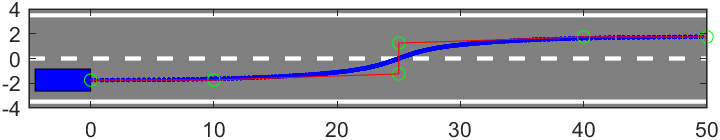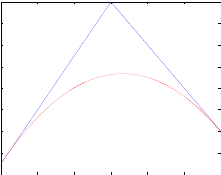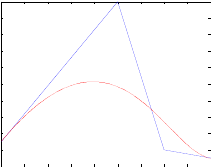• MATLAB-3次B样条
千次阅读
2020-12-24 11:35:11

High-speed，high-precision and high-efficiency A1-CNC．

MATLAB 样条工具箱可以通过节点获得样本函数值，但不能根据x求y或z，也不能求得样本曲线方程。例如：

ctrlpoints=[

0 -1.2 -1.6 -1.4 -1 -0.5 -0.35 -0.6 -1.6

-0.2 -0.5 -1 -1.5 -2.2 -2.7 -3.2 -3.7 -4.2];

knots=[0 0 0 0 1 2 3 4 5 6 6 6 6];

x0=ctrlpoints(1,:);

y0=ctrlpoints(2,:);

plot(x0,y0,'ro');%画所有控制点

hold on;

sp = spmak(knots,ctrlpoints);%生成B样条函数

fnplt(sp,[knots(1),knots(13)]);%根据所有节点，画样条曲线图

%fnplt(sp,'b*');

%hold on;

dt=knots(1):1:knots(13);

p = fnval(sp,dt);%计算在给定点处的样条函数值

plot(p(1,:),p(2,:),'*g');

dp1 = fnder(sp);%求样条函数的微分(即求导数)

dp = fnval(dp1,dt);

len = size(dt,2);

for i = 1:len

dir = dp(:,i);

scale = 1/sqrt(dir(1)^2+dir(2)^2);

dir = dir*scale;

plot([p(1,i),p(1,i)+dir(2)],[p(2,i),p(2,i)-dir(1)],'r');

end

axis equal

------------------------------------------------------------------

另外：

Matlab 样条工具箱(Spline ToolBox)

Matlab样条工具箱中的函数提供了样条的建立，操作，绘制等功能；

一. 样条函数的建立

第一步是建立一个样条函数，曲线或者曲面。这里的样条函数，根据前缀，分为4类：

cs* 三次样条

pp* 分段多项式样条，系数为t^n的系数

sp* B样条， 系数为基函数B_n^i(t)的系数

rp* 有理B样条

二. 样条操作

样条操作包括：函数操作：求值，算术运算，求导求积分等等

节点操作：主要是节点重数的调节，设定，修改等等

三. 简单示例

% step1: load ctrlpoints and knots

% step 2: create the spline

sp = spmak(knots,ctrlpoints');

fnplt(sp,[knots(5),knots(42)]);

% step 3: get points on the curve

dt = knots(5):3:knots(42);

p = fnval(sp,dt);

plot(p(1,:),p(2,:),'.g')

% step 4: get dir draw normals

dp1 = fnder(sp);

dp = fnval(dp1,dt);

len = size(dt,2);

for i = 1:len

dir = dp(:,i);

scale = 1/sqrt(dir(1)^2+dir(2)^2);

dir = dir*scale;

plot([p(1,i),p(1,i)+dir(2)],[p(2,i),p(2,i)-dir(1)],'r');

end

附：样条工具箱函数

1. 三次样条函数

csapi 插值生成三次样条函数

csape 生成给定约束条件下的三次样条函数

csaps 平滑生成三次样条函数

cscvn 生成一条内插参数的三次样条曲线

getcurve 动态生成三次样条曲线

2. 分段多项式样条函数

ppmak 生成分段多项式样条函数

ppual 计算在给定点处的分段多项式样条函数值

3. B样条函数

spmak 生成B样条函数

spcrv 生成均匀划分的B样条函数

spapi 插值生成B样条函数

spap2 用最小二乘法拟合生成B样条函数

spaps 对生成的B样条曲线进行光滑处理

spcol 生成B样条函数的配置矩阵

4. 有理样条函数

rpmak 生成有理样条

更多相关内容
• 双三次B样条曲面生成算法实现的matalb程序，有结果图片
• 早期工程师制图时，把富有弹性的细长木条(所谓样条)用压铁固定在点上，在其他地方让它自由弯曲，然后沿木条画下曲线。对于样条本身，可以利用材料力学的大柔度梁理论建立梁的挠度方程，根据理论，样条可以用分段...

matlab三次样条函数的绘制(spline和csape函数详解)

样条函数是工程中常用的插值函数。早期工程师制图时，把富有弹性的细长木条(所谓样条)用压铁固定在样点上，在其他地方让它自由弯曲，然后沿木条画下曲线。对于样条本身，可以利用材料力学的大柔度梁理论建立梁的挠度方程，根据理论，样条可以用分段插值三次函数表示。

由于样条曲线具有连续的二阶导数，所以光滑性好。matlab里有两个函数可以绘制样条曲线，一个是spline，一个是csape。虽然interp1中也可以绘制，优点是代码简单而且可以方便更换其它插值方法，但是功能也比较简单，对于边界条件的无法设置。

关于interp1可以参见官方帮助https://ww2.mathworks.cn/help/matlab/ref/interp1.html

三次样条曲线有4种边界条件。

自然边界条件，二阶导数在边界处为0，可视为简支梁，是最常用的边界条件。

第一边界条件，二阶导在边界处已知的边界条件。自然边界条件可视为特例。

第二边界条件，一阶导在边界处已知的边界条件。

循环边界条件，一阶导与二阶导在边界处相等的边界条件，适用于封闭或循环的图形。

如果既有第一边界由于第二边界，称为混合边界条件。

1.spline函数详解

spline函数只能实现自然边界条件和第二边界条件，可以实现一维或者高维的曲线插值。

官方spline实例可以参见：https://ww2.mathworks.cn/help/matlab/ref/spline.html

1.一维自然边界条件

格式cs=spline(x,y);

输入：x自变量，y函数值

输出：cs为三维样条插值函数构建的结构体。

cs结构体调用方法为yy=ppval(cs,xx);

xx为插值点，yy为插值得到的函数值。

%正弦曲线

r=pi*linspace(-1,1,100);

yr=sin(xr);

%1自然边界条件

x = pi*linspace(-1,1,5);%设置5个控制点

y = sin(x);

cs = spline(x,y);%样条函数

xx = linspace(x(1),x(end),100);%插值点

yy=ppval(cs,xx);%插值

figure(1)

plot(x,y,'bo',xr,yr,'b--',xx,yy,'r-');%绘图这里自然条件下，y’’(-pi)=y’’(pi)=0。红线为插值函数，蓝线为实际正弦函数。

2.第二边界条件

曲线依然选用上面的曲线，我们使用第二边界条件。

这里依然用上一个xr和yr正弦曲线。y’(-pi)=1，y’(pi)=0。

第二边界条件(导数条件)格式为：

cs=spline(x , [y’1, y, y’2]);

当y比x的长度多2个时，把第一个值和最后一个值当做函数边界的导数。

比如下面代码中，这里x有5个值，y在spline函数中在第一和最后分别增加了1个值作为导数值，第一个值为1，对应y’(-pi)=1；最后一个值为0，对应y’(pi)=0。

%2第二种边界条件，导数确定的

cs2 = spline(x,[1,y,0]);

xx = linspace(x(1),x(end),100);

yy=ppval(cs2,xx);

figure(2)

plot(x,y,'bo',xr,yr,'b--',xx,yy,'r-');可以看到插值函数被大大改变。

如果想要验证y’(-pi)=1，取(yy(2)-yy(1))/(xx(2)-xx(1))，得0.87(这里不是1的原因是xx太稀疏，xx间隔取越密，越接近1)。

3.高维无约束

这里以二维为例。这里选用两端无约束的自然边界条件。

二维格式为:

cs=spline(t,XY);

输入t为一个正向序列，需要单调。

输入XY为2行n列的矩阵，第一行为x，第二行为y。

cs依然是输出的结构体，但是后面利用ppval插值时要代入关于t的序列值。

XY=[1.1,1,0,-1,0,1,1.1;

2,1,2,0,-2,-1,-2];

t=1:length(XY);%输入XY和t

cs3=spline(t,XY);%样条函数

yy=ppval(cs3,linspace(t(1),t(end),101));%插值

figure(3)

plot(XY(1,:),XY(2,:),'bo',yy(1,:),yy(2,:))4.高维第二边界

由于1维不能表述当y’(x)=inf的情况(如果输入inf时函数会报错)，所以可以利用二维来实现导数为无穷大时的约束。

对于二维情况，导数的表达用向量的形式代替，比如k=1可以表示为(0.707,0.707)，k=∞可以用(0,1)表示。

表达格式为

cs=spline(t , [xy’1, xy, xy’2]);

t为序列

xy为坐标，xy’1为第一个点的导数列向量，xy’2为最后一个点的导数列向量

这里第一个点和最后一个点都取负无穷(0 ; -1)

%4高维有斜率约束

%这里用3的t和XY

cs4=spline(t,[[0;-1],XY,[0;-1]]);

yy=ppval(cs4,linspace(t(1),t(end),101));

figure(4)

plot(XY(1,:),XY(2,:),'bo',yy(1,:),yy(2,:))可以看到有负无穷约束的条件下，改善了图形向外弯折。

5.利用第二边界条件绘制圆

虽然圆是一个封闭图形，需要用到循环边界条件，但是如果我们已知圆一点处的导数，还是可以间接的利用这点的导数去做一个伪循环条件的。

比如在(1,0)点处作为初始点，逆时针一圈回到(1,0)点。两边一阶导均为正无穷。算法实现如下：

%5圆形绘制

t = pi*linspace(0,2,7);

XY=[cos(t);sin(t)];

cs5=spline(t,[[0;1],XY,[0;1]]);

yy=ppval(cs5,linspace(t(1),t(end),101));

figure(5)

plot(XY(1,:),XY(2,:),'bo',yy(1,:),yy(2,:))2.csape函数详解

csape函数可以实现三次样条曲线的各种条件，包括第一边界、第二边界、循环边界、混合边界等。可以实现一维至多维的各种情况。

1.自然边界条件

调用格式同spline函数，这里就不展开了。

cs =csape(x,y)

2。第一边界条件

调用格式：

cs = csape(x , [y’‘1 , y , y’‘2] , [2 , 2] )

其中x、y和y’'1(2)和之前定义都一样，是约束值，只是这个函数多了一项。

第三项，即[2,2]代表第一个值和最后一个值，都是样条函数的2阶导数值约束条件。

举例为

%采用cs1的x和y值，即正弦曲线值

cs6 = csape(x,[5,y,5],[2,2]);%样条插值

xx = linspace(x(1),x(end),100);

yy=ppval(cs6,xx);

figure(6)

plot(x,y,'bo',xr,yr,'b--',xx,yy,'r-');3.第二边界条件&混合边界条件

这里第三项取[1,1]即为第二边界条件，即1阶导数约束点。

如果取[1,2]或[2,1]即为混合边界条件。

这里仅用第二边界举例

cs7 = csape(x,[-1,y,-1],[1,1]);

xx = linspace(x(1),x(end),100);

yy=ppval(cs7,xx);

figure(7)

plot(x,y,'bo',xr,yr,'b--',xx,yy,'r-');可以看到和之前用二阶导等于0约束相比，用一阶导都等于-1的精度要更高。

4.二维循环边界条件

这里csape函数高维的调用格式依然同spline，具体参见前面的例子。

这里第三项为[0,0]时，代表循环边界条件，不仅适用于1维，还适用于更高维。

比如还是那绘制圆形举例：

%9二维自然条件

t = pi*linspace(0,2,5);

XY=[cos(t);sin(t)];

cs9=csape(t,XY);

yy=ppval(cs9,linspace(t(1),t(end),101));

figure(9)

plot(XY(1,:),XY(2,:),'bo',yy(1,:),yy(2,:))

axis equal

%10二维循环

cs10=csape(t,XY,[0,0]);%只多了第三项

yy=ppval(cs10,linspace(t(1),t(end),101));

figure(10)

plot(XY(1,:),XY(2,:),'bo',yy(1,:),yy(2,:))在(1,0)点自然无约束条件↑↑↑。在(1,0)点循环约束↑↑↑。

展开全文• B样条曲线_原理 &用于路径规划的matlab代码

B样条曲线在贝塞尔曲线的基础上有了更进一步的提高。

一 由于贝塞尔曲线存在以下不足：

1 ）缺乏局部修改性，即改变某一控制点对整个曲线都有影响。

2 ）n 较大时，特征多边形的边数较多，对曲线的控制减弱。

3 ）幂次过高难于修改。（而在外形设计中，局部修改是随时要进行的）。

二 所以提出了B样条曲线，B样条曲线有以下优点：

1 ）逼近特征多边形的精度更高。

2 ）多边形的边数与基函数的次数无关。

3 ）具有局部修改性。
B样条曲线的原理学习可参考下边链接，内容丰富，步骤精细。

https://www.cnblogs.com/icmzn/p/5100761.html

用于路径规划的matlab代码：

1.B_spline_curve.m 主函数

%% 三次B样条曲线
clc;
clear;
close all;

%% 数据定义
% 6个控制点
P=[0 10 25 25 40 50;
% 从0开始算 6个控制点 n=5
n=size(P,2)-1;
% K阶 也就是K-1次B样条
k=4;
path=[];
Bik=zeros(n+1,1);
flag=2;
%% 准均匀B样条
if flag==2
NodeVector=unEven(n,k-1);
for u=0:0.005:1-0.005
for i=0:1:n
Bik(i+1,1)=BaseFunction(i,k-1,u,NodeVector);
end
p_u=P*Bik;
path=[path;[p_u(1,1),p_u(2,1)]];
end
elseif flag==1
%% 均匀B样条
NodeVector=linspace(0,1,n+k+1);
for u=(k-1)/(n+k+1):0.001:(n+2)/(n+k+1)
for i=0:1:n
Bik(i+1,1)=BaseFunction(i,k-1,u,NodeVector);
end
p_u=P*Bik;
path=[path;[p_u(1,1),p_u(2,1)]];
end
end
%% 绘图
% 车宽 车长 路长
car_width=1.8;
car_length=4.5;
%灰色路面区域设置 填充 车辆位置填充
hold on
fill([0 0 -car_length -car_length],...

% 绘制道路中间虚线 两侧实线

% 横纵坐标长度设置
axis equal

scatter(path(:,1),path(:,2),'.b');%绘制路径散点
scatter(P(1,:),P(2,:),'g');
plot(P(1,:),P(2,:),'r');

2.BaseFunction.m 基函数的递归运算.

function Bik_u = BaseFunction(i,k,u,NodeVector)
%基函数    Bik

if k==0
if u>= NodeVector(i+1) && u<NodeVector(i+2)
Bik_u = 1;
else
Bik_u = 0;
end
else
Length1=NodeVector(i+k+1)-NodeVector(i+1);
Length2=NodeVector(i+k+2)-NodeVector(i+2);
if Length1 == 0
Length1=1;
end
if Length2 == 0
Length2 = 1;
end
% 递归 Bik_u=（u-u_i）/(u_i+k-u_i)*B_i_k-1_u
%             +(u_i+k+1-u)/(u_i+k+1-u_i+1)* B_i+1_k-1_u
Bik_u=(u-NodeVector(i+1))/Length1 * BaseFunction(i,k-1,u,NodeVector)...
+(NodeVector(i+k+2)-u)/Length2 * BaseFunction(i+1,k-1,u,NodeVector);
end



3.unEven.m 准均匀B样条的节点向量生成

节点向量前面和后面各有k+1个重复值 前面是K个0 后面是K个1

function NodeVector = unEven(n,k)
%% 准均匀B样条曲线节点向量计算。传入的n从0开始，所以共n+1个控制顶点。k阶曲线为k-1次。节点向量个数为n+1+k+1=n+k+2
NodeVector=zeros(1,n+k+2);

piecewise=n-k+1;     %曲线段数
if piecewise == 1    %只有一条曲线时 n=k
for i=k+2 : n+k+2
NodeVector(1,i)=1;
end
else   %不止一条曲线
flag=1;
while flag ~= piecewise
NodeVector(1,k+flag+1) = NodeVector(1,k+flag) + 1 /piecewise;
flag=flag+1;
end
NodeVector(1,n+2:n+k+2)=1;       %节点向量前面和后面各有k+1个重复值 前面是K个0 后面是K个1

end



准均匀的B样条效果图展开全文自动驾驶
• 二次Bezier曲线代码：m-文件函数：function bezier2(p0,p1,p2)t=0:0.001:1;x=(p2(1)-2*p1(1)+p0(1))*t.^2+2*(p1(1)-p0(1))*t+p0(1);y=(p2(2)-2*p1(2)+p0(2))*t.^2+2*(p1(2)-p0(2))*t+p0(2);plot([p0(1) p1(1) p2(1)]...

二次Bezier曲线代码：

m-文件函数：

function bezier2(p0,p1,p2)

t=0:0.001:1;

x=(p2(1)-2*p1(1)+p0(1))*t.^2+2*(p1(1)-p0(1))*t+p0(1);

y=(p2(2)-2*p1(2)+p0(2))*t.^2+2*(p1(2)-p0(2))*t+p0(2);

plot([p0(1) p1(1) p2(1)],[p0(2) p1(2) p2(2)],'b'),hold on

plot(x,y,'r');

执行：

>> bezier2([1,3],[4,18],[7,6])三次Bezier曲线代码：

function bezier3(p0,p1,p2,p3)

t=0:0.001:1;

x=(1-t).^3*p0(1)+3*t.*(1-t).^2*p1(1)+3*t.^2.*(1-t)*p2(1)+t.^3*p3(1); y=(1-t).^3*p0(2)+3*t.*(1-t).^2*p1(2)+3*t.^2.*(1-t)*p2(2)+t.^3*p3(2); plot([p0(1) p1(1) p2(1) p3(1)],[p0(2) p1(2) p2(2) p3(2)],'b');

hold on;

plot(x,y,'r');

执行：

>> bezier3([0,3],[5,20],[7,2],[9,1])B样条曲线代码(方法一)：

展开全文• 展开全部看看这个能不能帮到你：Matlab中插值62616964757a686964616fe4b893e5b19e31333339666135函数汇总和使用说明:MATLAB中的插值函数为interp1，其调用格式为：yi=interp1(x,y,xi,'method')其中x，y为插值点，yi...
• 由于毕业设计的需要，生成的刀位点，个人觉得间隔有点大，为了能够以后用于实际加工，因此花了一上午的时间稍微了解了一下B样条曲线，废话不多说，直接上代码 import matplotlib as mpl import matplotlib.pyplot as...python
• 这就需要进行带时间参数的轨迹规划处理，也就是对这空间轨迹进行速度、加速度约束，并且计算运动到每个路点的时间，高级的算法有TOPP等，一般的呢就是贝塞尔、次准/非/均匀B、五次及次样条等。下面从最简单的...
• 本文为路径规划和matlab的学习随笔，主要参考 matlab帮助文档里的“Path Following for a Differential Drive Robot”、大学生mooc“自主移动机器人”和哔哩哔哩：...
• ## MATLAB常用算法

热门讨论 2010-04-05 10:34:28
BSample 求已知数据点的第一类B样条的插值 DCS 用倒差商算法求已知数据点的有理分式形式的插值分式 Neville 用Neville算法求已知数据点的有理分式形式的插值分式 FCZ 用倒差商算法求已知数据点的有理分式形式的插值...
• BSample 求已知数据点的第一类B样条的插值 DCS 用倒差商算法求已知数据点的有理分式形式的插值分式 Neville 用Neville算法求已知数据点的有理分式形式的插值分式 FCZ 用倒差商算法求已知数据点的有理分式形式的插值...
• 维地图定义，散点拟合插值与粒子群算法matlab实现） 本文基于小黎的ally学习资料实现，感谢该博主的无私奉献 维地图与二维地图的差别 1.二维无高度信息，多用于地面机器人，自动驾驶 2.二维地图路径规划有：全...
• 【实例简介】包括了全书所有实例对应的MATLAB的M文件。所有代码按照章节存放在各个文件夹下，如“第4章插值”文件夹下存放了本书第4章所有的算法程序代码，对于算法的程序代码，在光盘中存为同函数名的M文件，例如，...
• 文章目录前言一、MATLAB是什么？二、常用函数1.MATLAB数据类型2.读入数据总结 前言 这是一篇讲解了MATLAB常用函数的文章，希望对您有所帮助。 一、MATLAB是什么？   MATLAB是美国MathWorks公司出品的商业数学...矩阵
• 自动驾驶中轨迹规划是最常见的问题，本文分以下几个... 自动驾驶中经常遇到一类问题，要把一轨迹线（离散点），拟合成一多项式表达形式： 首先看不带约束的多项式拟合（最小二乘法）： 误差函数： 为了求...
• 曲线拟合数值方法求解，主要是...然后，分析各算法流程和核心计算代码模块组织结构，然后对各算法的实例进行计算分析、绘图显示与数据表格结果显示，最后对并对曲线拟合的数值计算方法的各算法特点以及优缺点进行总结。机器学习
• ## 二维曲线拟合

千次阅读 2018-11-20 16:49:03
EVD）Householder变换法（QR Factorization,QR分解）奇异值分解（singular value decomposition,SVD）Matlab样条工具箱(Curve Fitting Toolbox)与曲线拟合导入数据，打开工具箱选择合适的模型...
• 分别用B样条函数对y和f(x)中的自选数据进行5次B样条函数拟合，并与次分段多项式样条函数拟合的结果相比较。 解： MATLAB代码如下： clc;clear; %%y函数部分 x0=[0,0.4,1,2,pi]; y0=sin(x0); ezplot('sin(t)...多项式
• 01线性回归线性模型损失函数目标：找到θ使损失函数最小用标准方程式直接计算θ最优解标准方程推导过程随机生成一些线性数据用标准方程式求最优参数用LinearRegression求解标准方程计算复杂度,数据越多越慢线性方程...
• 基于matlab的图像形状与分类摘 要数字图像处理是一门新兴技术，随着计算机硬件的发展，数字图像的实时处理已经成为可能，由于数字图像处理的各种算法的出现，使得其处理速度越来越快，能更好的为人们服务。...计算机视觉 机器学习 人工智能 编程语言
• matlab的多元函数微积分学.ppt》由会员分享，可在线阅读，更多相关《matlab的多元函数微积分学.ppt(36页珍藏版)》请在人人文库网上搜索。1、2.3 多元函数微积分学,2.3.1 多元函数的微分,1、符号求导指令diff 多元...
• } 运动模型的离散积分-欧拉法 运动学模型模型线性化-离散化 https://zhuanlan.zhihu.com/p/156822207 运动模型的离散积分－中值法 B-Spline 产生 IMU数据 B样条曲线（B-spline Curves） Bezier曲线有以下几个不足点...ORB_SLAM3
• Floyd 感觉大多都能用 floyd 解决 % 这是floyd算法，以下注释为渡边所加 % floyd不能解决多最短路径和负权回路的问题 % 解稠密图效果最佳，边权可正可负，是双源解法 % 对于稠密图，效率要高于执行|V|次Dijkstra...
• 文章目录简介一、介绍二、原理飞行走廊生成三、项目演示参考 简介 Btraj是一个在线的四旋翼无人机的运动规划框架，主要用于未知环境中的自主导航。基于机载状态估计和环境感知，采用基于快速行进（FM *）的路径搜索...
• 广告关闭腾讯云11.11云上盛惠 ，精选热门...利用python生成曲线图像的脚本，参考自：http:www.lorenzogil.comprojectspychaimportcairoimportpycha.barwidth,height=500,400surface=cairo.imagesurface(cairo.forma...
• end 1.5 样条插值 1.5.1 样条函数的概念 1.5.2 二次样条函数插值 1.5.3 次样条函数插值 1.5.4 次样条插值在 Matlab 中的实现 Matlab次样条插值也有现成的函数： y=interp1(x0,y0,x,‘spline’)；...
• 9. NUBUS五次非均匀B样条轨迹规划及机械臂仿真 10.五次&次多项式连续轨迹规划 11. 主从机械臂||双机械臂跟随控制 1.六自由度机械臂逆解仿真 内容简介： 六自由度机械臂逆运动学仿真，通过给定圆形轨迹，...
• 由于最近新参与了一个与智能优化相关的科研课题，所以需要了解一些计算智能的算法知识。经老师推荐，从网上购买了张军老师的这本《计算智能》，尽管出版年份距今已有一些年日，但因该领域多年来并未有太多新的大改变...人工智能...

# 三阶b样条曲线生成算法matlabmatlab 订阅